# Subtraction: Whole Numbers

Subtraction , written using the symbol $-$ ,   is the mathematical operation that represents the number of objects left in a collection after some number is removed.

For simple subtraction problems, you can count dots to find the answer.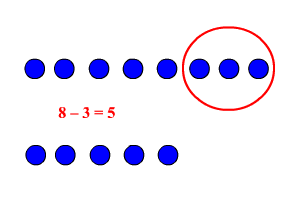If you start with $8$ dots, and then take away $3$ dots (in the circle), you are left with $5$ dots.

To subtract numbers with more than one digit, you can visualize base ten blocks.

$94-78$

$94$ is equal to $90+4$ , so it can be represented by NINE ten blocks and FOUR unit blocks.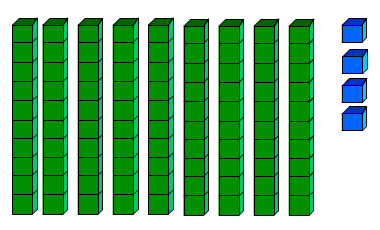$78$ is equal to $70+8$ , so it can be represented by SEVEN ten blocks and EIGHT unit blocks. This is the number we want to take away.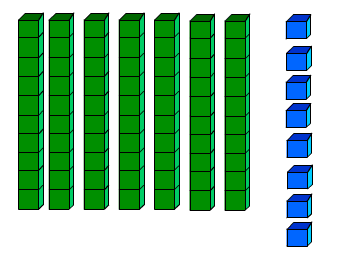We don't have enough unit blocks in the first set to take away $8$ . So we have to break up one ten block.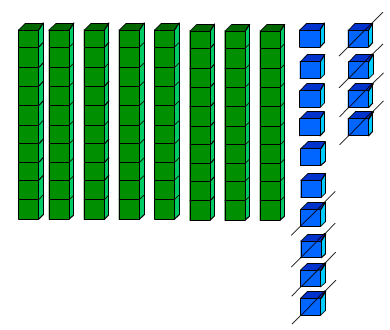Now we can take away $8$ unit blocks and $7$ ten blocks. We are left with $1$ ten block and $6$ unit blocks, or $10+6=16$ .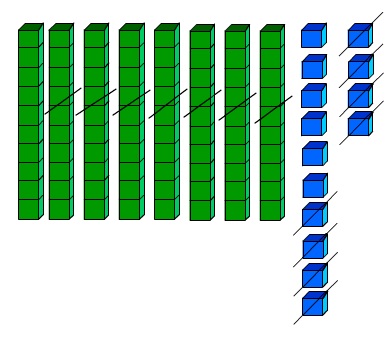So, $94-78=16$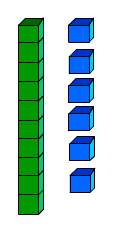When you subtract by writing numbers vertically, and subtracting column-by-column, you use "borrowing", which is the same as the idea of breaking up one of the next-largest blocks.

$\begin{array}{l}\text{\hspace{0.17em}}\text{\hspace{0.17em}}\text{\hspace{0.17em}}3053\\ \underset{_}{-\text{\hspace{0.17em}}\text{\hspace{0.17em}}737}\end{array}$

First subtract the ones column. Since $3$ is smaller than $7$ , borrow $1$ ten from $5$ and then regroup into ones ( $10+3=13$ ). Hence $10+3=13$ .

$\begin{array}{l}\text{\hspace{0.17em}}\text{\hspace{0.17em}}3\text{\hspace{0.17em}}0\stackrel{{4}}{\overline{)5}}\stackrel{{13}}{\overline{)3}}\\ \underset{_}{-\text{\hspace{0.17em}}\text{\hspace{0.17em}}7\text{\hspace{0.17em}}\text{\hspace{0.17em}}3\text{\hspace{0.17em}}\text{\hspace{0.17em}}7}\\ \text{\hspace{0.17em}}\text{\hspace{0.17em}}\text{\hspace{0.17em}}\text{\hspace{0.17em}}\text{\hspace{0.17em}}\text{\hspace{0.17em}}\text{\hspace{0.17em}}\text{\hspace{0.17em}}\text{\hspace{0.17em}}\text{\hspace{0.17em}}\text{\hspace{0.17em}}\text{\hspace{0.17em}}\text{\hspace{0.17em}}\text{\hspace{0.17em}}\text{\hspace{0.17em}}{6}\end{array}$

Next subtract the tens column. $4-3=1$

$\begin{array}{l}\text{\hspace{0.17em}}\text{\hspace{0.17em}}3\text{\hspace{0.17em}}0\stackrel{{4}}{\overline{)5}}\stackrel{{13}}{\overline{)3}}\\ \underset{_}{-\text{\hspace{0.17em}}\text{\hspace{0.17em}}7\text{\hspace{0.17em}}\text{\hspace{0.17em}}3\text{\hspace{0.17em}}\text{\hspace{0.17em}}7}\\ \text{\hspace{0.17em}}\text{\hspace{0.17em}}\text{\hspace{0.17em}}\text{\hspace{0.17em}}\text{\hspace{0.17em}}\text{\hspace{0.17em}}\text{\hspace{0.17em}}\text{\hspace{0.17em}}\text{\hspace{0.17em}}\text{\hspace{0.17em}}{1}\text{\hspace{0.17em}}\text{\hspace{0.17em}}6\end{array}$

Next subtract the hundreds column. Since $0$ is smaller than $7$ , borrow $1$ ten hundred from $3$ and then regroup into hundred . Hence $10-7=3$ .

$\begin{array}{l}\text{\hspace{0.17em}}\stackrel{{2}}{\overline{)3}}\stackrel{{10}}{\overline{)0}}\stackrel{{4}}{\overline{)5}}\stackrel{{13}}{\overline{)3}}\\ \underset{_}{-\text{\hspace{0.17em}}\text{\hspace{0.17em}}\text{\hspace{0.17em}}\text{\hspace{0.17em}}\text{\hspace{0.17em}}7\text{\hspace{0.17em}}\text{\hspace{0.17em}}\text{\hspace{0.17em}}3\text{\hspace{0.17em}}\text{\hspace{0.17em}}\text{\hspace{0.17em}}7}\\ \text{\hspace{0.17em}}\text{\hspace{0.17em}}\text{\hspace{0.17em}}\text{\hspace{0.17em}}\text{\hspace{0.17em}}\text{\hspace{0.17em}}\text{\hspace{0.17em}}\text{\hspace{0.17em}}{3}\text{\hspace{0.17em}}\text{\hspace{0.17em}}\text{\hspace{0.17em}}1\text{\hspace{0.17em}}\text{\hspace{0.17em}}\text{\hspace{0.17em}}6\end{array}$

Next subtract the thousands column. $2-0=2$ .

$\begin{array}{l}\text{\hspace{0.17em}}\stackrel{{2}}{\overline{)3}}\stackrel{{10}}{\overline{)0}}\stackrel{{4}}{\overline{)5}}\stackrel{{13}}{\overline{)3}}\\ \underset{_}{-\text{\hspace{0.17em}}\text{\hspace{0.17em}}\text{\hspace{0.17em}}\text{\hspace{0.17em}}\text{\hspace{0.17em}}7\text{\hspace{0.17em}}\text{\hspace{0.17em}}\text{\hspace{0.17em}}3\text{\hspace{0.17em}}\text{\hspace{0.17em}}\text{\hspace{0.17em}}7}\\ \text{\hspace{0.17em}}\text{\hspace{0.17em}}\text{\hspace{0.17em}}{2}\text{\hspace{0.17em}}\text{\hspace{0.17em}}\text{\hspace{0.17em}}3\text{\hspace{0.17em}}\text{\hspace{0.17em}}\text{\hspace{0.17em}}1\text{\hspace{0.17em}}\text{\hspace{0.17em}}6\end{array}$

Unlike addition, for real numbers , the subtraction operation is not commutative . That is, order matters:

$\begin{array}{l}94-78=16\\ 7-3=4\end{array}$

but $3-7=-4$ , a negative value.